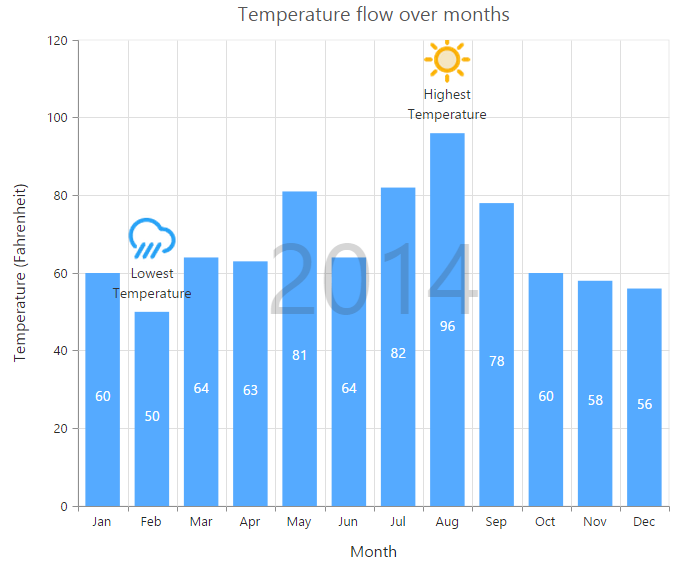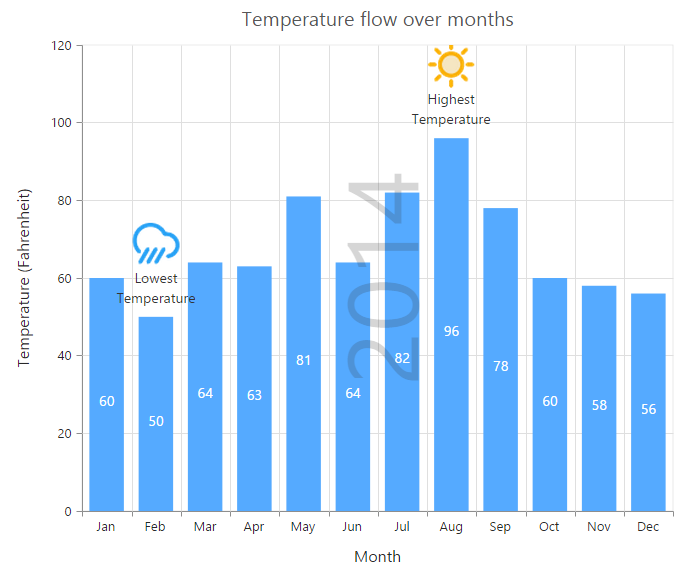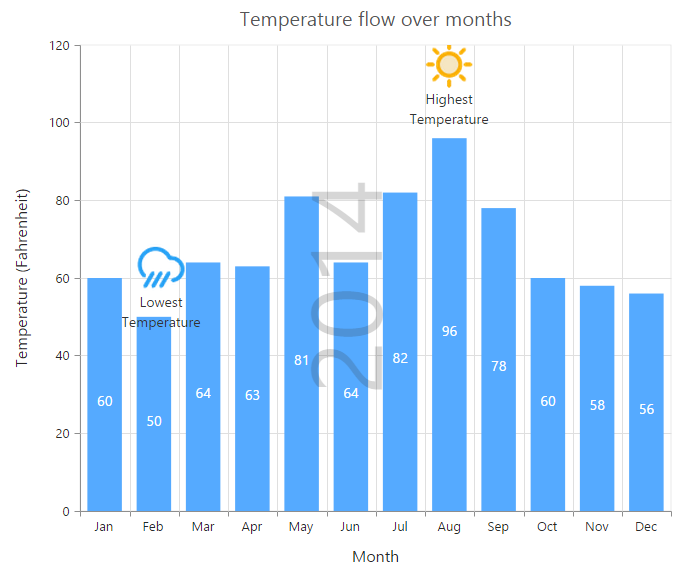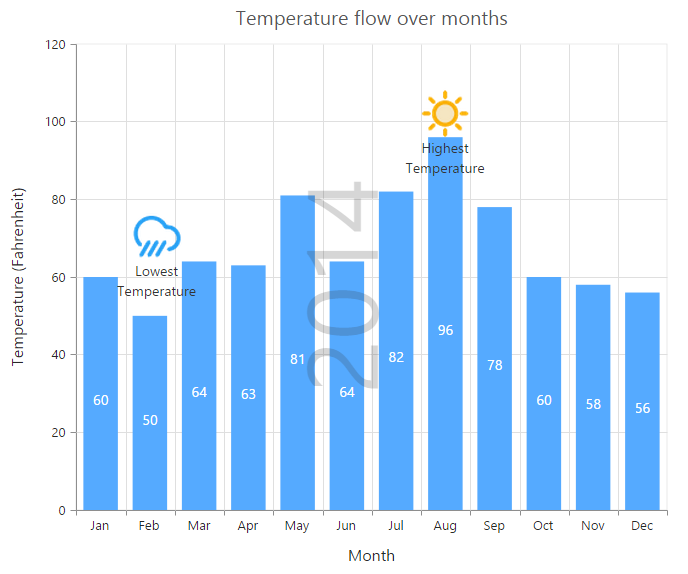# Annotations

8 Jun 20173 minutes to read

annotations are used to mark the specific area of interest in the chart area with texts, shapes or images.

You can add annotations to the chart by using the `annotations` option. By using the `content` option of annotation object, you can specify the id of the element that needs to be displayed in the chart area.

• HTML
• ``````<div id= "watermark" style="font-size:100px; display:none">2014</div>

<ej-chart id="chartcontainer">
<e-annotations>
<e-annotation [visible]="true" content="watermark" [opacity]=0.2 region="series">
</e-annotation>
</e-annotations>
</ej-chart>``````## Rotate the annotation template

To rotate the annotation template, you can use the `angle` property of the annotations.

• HTML
• ``````<ej-chart id="chartcontainer">
<e-annotations>
<e-annotation [visible]="true" content="watermark" [opacity]=0.2
region="series" [angle]="270">
</e-annotation>
</e-annotations>
</ej-chart>``````## Positioning Annotation

You can position annotations either by using the coordinates (`x` and `y` options) or by using the alignment options (`horizontalAlignment` and `verticalAlignment`).

By using the `coordinateUnit` option, you can specify whether the value provided in the `x` and `y` options are relative to the chart or axis.

• If the coordinateUnit is set to none, the annotations are placed relative to the chart/plot area by using the `horizontalAlignment` and `verticalAlignment` options.

• If the coordinateUnit is set to points, the x and y values of the annotation are the coordinates relative to the axis and annotation is positioned relative to the axis. By default, the x and y values are associated with the [`primaryXAxis`] and [`primaryYAxis`]. In case, when the chart contains multiple axis and you want to associate the annotation with a particular axis, you can specify the `xAxisName` and `yAxisName` options of the annotation object.

• If the coordinateUnit is set to pixels, the x and y values are coordinates relative to the top-left corner of the chart/plot area.

NOTE

By using the `region` option, you can specify whether the annotation is placed relative to the entire chart or plot area.

• HTML
• ``````<ej-chart id="chartcontainer">
<e-annotations>
<!--Change coordinateUnit type to pixels-->
<e-annotation [visible]="true" content="lowtemp" coordinateUnit="pixels"
[x]="170" [y]="350">
</e-annotation>
</e-annotations>
</ej-chart>``````## Annotation alignments

When the coordinateUnit is set to pixels or points, you can align the annotation relative to the coordinates by using the `horizontalAlignment` and [`verticalAlignment`] options.

• HTML
• ``````<ej-chart id="chartcontainer">
<e-annotations>
<!--Change alignment of annotation template-->
<e-annotation [visible]="true" content="lowtemp" verticalAlignment="middle"
horizontalAlignment="far" margin-right="40">
</e-annotation>
</e-annotations>
</ej-chart>``````